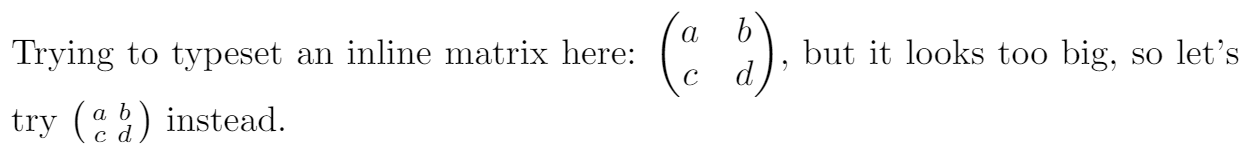Aller au contenu

## amsmath matrix environments

The amsmath package provides commands to typeset matrices with different delimiters. Once you have loaded \usepackage{amsmath} in your preamble, you can use the following environments in your math environments:

Type LaTeX markup Renders as
Plain \begin{matrix}1 & 2 & 3\\a & b & c\end{matrix} $$\begin{matrix}1 & 2 & 3\\a & b & c\end{matrix}$$
Parentheses;
round brackets
\begin{pmatrix}1 & 2 & 3\\a & b & c\end{pmatrix} $$\begin{pmatrix}1 & 2 & 3\\a & b & c\end{pmatrix}$$
Brackets;
square brackets
\begin{bmatrix}1 & 2 & 3\\a & b & c\end{bmatrix} $$\begin{bmatrix}1 & 2 & 3\\a & b & c\end{bmatrix}$$
Braces;
curly brackets
\begin{Bmatrix}1 & 2 & 3\\a & b & c\end{Bmatrix} $$\begin{Bmatrix}1 & 2 & 3\\a & b & c\end{Bmatrix}$$
Pipes \begin{vmatrix}1 & 2 & 3\\a & b & c\end{vmatrix} $$\begin{vmatrix}1 & 2 & 3\\a & b & c\end{vmatrix}$$
Double pipes \begin{Vmatrix}1 & 2 & 3\\a & b & c\end{Vmatrix} $$\begin{Vmatrix}1 & 2 & 3\\a & b & c\end{Vmatrix}$$

If you need to create matrices with different delimiters, you can add them manually to a plain matrix. For example:

LaTeX markup Renders as
\left\lceil\begin{matrix}1 & 2 & 3\\a & b & c\end{matrix}\right\rceil $$\left\lceil\begin{matrix}1 & 2 & 3\\a & b & c\end{matrix}\right\rceil$$
\left\langle\begin{matrix}1 & 2 & 3\\a & b & c\end{matrix}\right\rvert $$\left\langle\begin{matrix}1 & 2 & 3\\a & b & c\end{matrix}\right\rvert$$
\left\langle\begin{matrix}1 & 2 & 3\\a & b & c\end{matrix}\right\rangle $$\left\langle\begin{matrix}1 & 2 & 3\\a & b & c\end{matrix}\right\rangle$$

## Inline matrices

When typesetting inline math, the usual matrix environments above may look too big. It may be better to use smallmatrix in such situations, although you will need to provide your own delimiters.

\documentclass{article}
\usepackage{amsmath}
\begin{document}
\noindent Trying to typeset an inline matrix here:
$\begin{pmatrix} a & b\\ c & d \end{pmatrix}$,
but it looks too big, so let's try
$\big(\begin{smallmatrix} a & b\\ c & d \end{smallmatrix}\big)$The mathtools package provides psmallmatrix, bsmallmatrix etc environments for convenience.